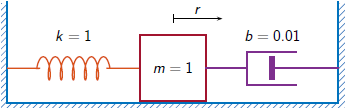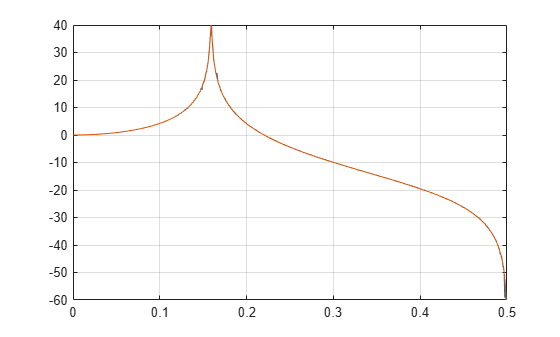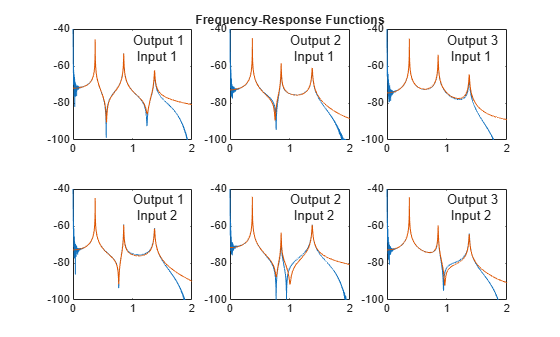# modalfit

Modal parameters from frequency-response functions

## Syntax

``fn = modalfit(frf,f,fs,mnum)``
``fn = modalfit(frf,f,fs,mnum,Name,Value)``
``````[fn,dr,ms] = modalfit(___)``````
``````[fn,dr,ms,ofrf] = modalfit(___)``````
``[___] = modalfit(sys,f,mnum,Name,Value)``

## Description

example

````fn = modalfit(frf,f,fs,mnum)` estimates the natural frequencies of `mnum` modes of a system with measured frequency-response functions `frf` defined at frequencies `f` and for a sample rate `fs`. Use `modalfrf` to generate a matrix of frequency-response functions from measured data. `frf` is assumed to be in dynamic flexibility (receptance) format.```

example

````fn = modalfit(frf,f,fs,mnum,Name,Value)` specifies additional options using name-value arguments.```

example

``````[fn,dr,ms] = modalfit(___)``` also returns the damping ratios and mode-shape vectors corresponding to each natural frequency in `fn`, using any combination of inputs from previous syntaxes.```

example

``````[fn,dr,ms,ofrf] = modalfit(___)``` also returns a reconstructed frequency-response function array based on the estimated modal parameters.```
````[___] = modalfit(sys,f,mnum,Name,Value)` estimates the modal parameters of the identified model `sys`. Use estimation commands like `ssest` (System Identification Toolbox) or `tfest` (System Identification Toolbox) to create `sys` starting from a measured frequency-response function or from time-domain input and output signals. This syntax allows use of the `'DriveIndex'`, `'FreqRange'`, and `'PhysFreq'` name-value arguments. It typically requires less data than syntaxes that use nonparametric methods. You must have a System Identification Toolbox™ license to use this syntax.```

## Examples

collapse all

Estimate the frequency-response function for a simple single-input/single-output system and compare it to the definition.

A one-dimensional discrete-time oscillating system consists of a unit mass, $\mathit{m}$, attached to a wall by a spring with elastic constant $\mathit{k}=1$. A sensor samples the displacement of the mass at ${\mathit{F}}_{\mathrm{s}}=1$ Hz. A damper impedes the motion of the mass by exerting on it a force proportional to speed, with damping constant $\mathit{b}=0.01$.Generate 3000 time samples. Define the sampling interval $\Delta \mathit{t}=1/{\mathit{F}}_{\mathit{s}}$.

```Fs = 1; dt = 1/Fs; N = 3000; t = dt*(0:N-1); b = 0.01;```

The system can be described by the state-space model

`$\begin{array}{c}x\left(k+1\right)=Ax\left(k\right)+Bu\left(k\right),\\ y\left(k\right)=Cx\left(k\right)+Du\left(k\right),\end{array}$`

where $\mathit{x}={\left[\begin{array}{cc}\mathit{r}& \mathit{v}\end{array}\right]}^{\mathit{T}}$ is the state vector, $\mathit{r}$ and $\mathit{v}$ are respectively the displacement and velocity of the mass, $\mathit{u}$ is the driving force, and $\mathit{y}=\mathit{r}$ is the measured output. The state-space matrices are

`$A=\mathrm{exp}\left({A}_{c}\Delta t\right),\phantom{\rule{1em}{0ex}}B={A}_{c}^{-1}\left(A-I\right){B}_{c},\phantom{\rule{1em}{0ex}}C=\left[\begin{array}{cc}1& 0\end{array}\right],\phantom{\rule{1em}{0ex}}D=0,$`

$\mathit{I}$ is the $2×2$ identity, and the continuous-time state-space matrices are

`${A}_{c}=\left[\begin{array}{cc}0& 1\\ -1& -b\end{array}\right],\phantom{\rule{1em}{0ex}}{B}_{c}=\left[\begin{array}{c}0\\ 1\end{array}\right].$`

```Ac = [0 1;-1 -b]; A = expm(Ac*dt); Bc = [0;1]; B = Ac\(A-eye(2))*Bc; C = [1 0]; D = 0;```

The mass is driven by random input for the first 2000 seconds and then left to return to rest. Use the state-space model to compute the time evolution of the system starting from an all-zero initial state. Plot the displacement of the mass as a function of time.

```rng default u = randn(1,N)/2; u(2001:end) = 0; y = 0; x = [0;0]; for k = 1:N y(k) = C*x + D*u(k); x = A*x + B*u(k); end plot(t,y)```Estimate the modal frequency-response function of the system. Use a Hann window half as long as the measured signals. Specify that the output is the displacement of the mass.

```wind = hann(N/2); [frf,f] = modalfrf(u',y',Fs,wind,'Sensor','dis');```

The frequency-response function of a discrete-time system can be expressed as the Z-transform of the time-domain transfer function of the system, evaluated at the unit circle. Compare the `modalfrf` estimate with the definition.

```[b,a] = ss2tf(A,B,C,D); nfs = 2048; fz = 0:1/nfs:1/2-1/nfs; z = exp(2j*pi*fz); ztf = polyval(b,z)./polyval(a,z); plot(f,20*log10(abs(frf))) hold on plot(fz*Fs,20*log10(abs(ztf))) hold off grid ylim([-60 40])```Estimate the natural frequency and the damping ratio for the vibration mode.

`[fn,dr] = modalfit(frf,f,Fs,1,'FitMethod','PP')`
```fn = 0.1593 ```
```dr = 0.0043 ```

Compare the natural frequency to $1/2\pi$, which is the theoretical value for the undamped system.

`theo = 1/(2*pi)`
```theo = 0.1592 ```

Compute the modal parameters of a Space Station module starting from its frequency-response function (FRF) array.

Load a structure containing the three-input/three-output FRF array. The system is sampled at 320 Hz.

```load modaldata SpaceStationFRF frf = SpaceStationFRF.FRF; f = SpaceStationFRF.f; fs = SpaceStationFRF.Fs;```

Extract the modal parameters of the lowest 24 modes using the least-squares rational function method.

`[fn,dr,ms,ofrf] = modalfit(frf,f,fs,24,'FitMethod','lsrf');`

Compare the reconstructed FRF array to the measured one.

```for ij = 1:3 for ji = 1:3 subplot(3,3,3*(ij-1)+ji) loglog(f,abs(frf(:,ji,ij))) hold on loglog(f,abs(ofrf(:,ji,ij))) hold off axis tight title(sprintf('In%d -> Out%d',ij,ji)) if ij==3 xlabel('Frequency (Hz)') end end end```Estimate the frequency-response function and modal parameters of a simple multi-input/multi-output system.

An ideal one-dimensional oscillating system consists of two masses, ${\mathit{m}}_{1}$ and ${\mathit{m}}_{2}$, confined between two walls. The units are such that ${\mathit{m}}_{1}=1$ and ${\mathit{m}}_{2}=\mu$. Each mass is attached to the nearest wall by a spring with an elastic constant $\mathit{k}$. An identical spring connects the two masses. Three dampers impede the motion of the masses by exerting on them forces proportional to speed, with damping constant $\mathit{b}$. Sensors sample ${\mathit{r}}_{1}$ and ${\mathit{r}}_{2}$, the displacements of the masses, at ${\mathit{F}}_{\mathrm{s}}=50$ Hz.Generate 30,000 time samples, equivalent to 600 seconds. Define the sampling interval $\Delta \mathit{t}=1/{\mathit{F}}_{\mathrm{s}}$.

```Fs = 50; dt = 1/Fs; N = 30000; t = dt*(0:N-1);```

The system can be described by the state-space model

`$\begin{array}{c}x\left(k+1\right)=Ax\left(k\right)+Bu\left(k\right),\\ y\left(k\right)=Cx\left(k\right)+Du\left(k\right),\end{array}$`

where $x={\left[\begin{array}{cccc}{r}_{1}& {v}_{1}& {r}_{2}& {v}_{2}\end{array}\right]}^{T}$ is the state vector, ${r}_{i}$ and ${v}_{i}$ are respectively the location and the velocity of the $i$th mass, $u={\left[\begin{array}{cc}{u}_{1}& {u}_{2}\end{array}\right]}^{T}$ is the vector of input driving forces, and $y={\left[\begin{array}{cc}{r}_{1}& {r}_{2}\end{array}\right]}^{T}$ is the output vector. The state-space matrices are

`$A=\mathrm{exp}\left({A}_{c}\Delta t\right),\phantom{\rule{1em}{0ex}}B={A}_{c}^{-1}\left(A-I\right){B}_{c},\phantom{\rule{1em}{0ex}}C=\left[\begin{array}{cccc}1& 0& 0& 0\\ 0& 0& 1& 0\end{array}\right],\phantom{\rule{1em}{0ex}}D=\left[\begin{array}{cc}0& 0\\ 0& 0\end{array}\right],$`

$\mathit{I}$ is the $4×4$ identity, and the continuous-time state-space matrices are

`${A}_{c}=\left[\begin{array}{cccc}0& 1& 0& 0\\ -2k& -2b& k& b\\ 0& 0& 0& 1\\ k/\mu & b/\mu & -2k/\mu & -2b/\mu \end{array}\right],\phantom{\rule{1em}{0ex}}{B}_{c}=\left[\begin{array}{cc}0& 0\\ 1& 0\\ 0& 0\\ 0& 1/\mu \end{array}\right].$`

Set $\mathit{k}=400$, $\mathit{b}=0.1$, and $\mu =1/10$.

```k = 400; b = 0.1; m = 1/10; Ac = [0 1 0 0;-2*k -2*b k b;0 0 0 1;k/m b/m -2*k/m -2*b/m]; A = expm(Ac*dt); Bc = [0 0;1 0;0 0;0 1/m]; B = Ac\(A-eye(4))*Bc; C = [1 0 0 0;0 0 1 0]; D = zeros(2);```

The masses are driven by random input throughout the measurement. Use the state-space model to compute the time evolution of the system starting from an all-zero initial state.

```rng default u = randn(2,N); y = [0;0]; x = [0;0;0;0]; for kk = 1:N y(:,kk) = C*x + D*u(:,kk); x = A*x + B*u(:,kk); end```

Use the input and output data to estimate the transfer function of the system as a function of frequency. Use a 15000-sample Hann window with 9000 samples of overlap between adjoining segments. Specify that the measured outputs are displacements.

```wind = hann(15000); nove = 9000; [FRF,f] = modalfrf(u',y',Fs,wind,nove,'Sensor','dis');```

Compute the theoretical transfer function as the Z-transform of the time-domain transfer function, evaluated at the unit circle.

```nfs = 2048; fz = 0:1/nfs:1/2-1/nfs; z = exp(2j*pi*fz); [b1,a1] = ss2tf(A,B,C,D,1); [b2,a2] = ss2tf(A,B,C,D,2); frf(1,:,1) = polyval(b1(1,:),z)./polyval(a1,z); frf(1,:,2) = polyval(b1(2,:),z)./polyval(a1,z); frf(2,:,1) = polyval(b2(1,:),z)./polyval(a2,z); frf(2,:,2) = polyval(b2(2,:),z)./polyval(a2,z);```

Plot the estimates and overlay the theoretical predictions.

```for jk = 1:2 for kj = 1:2 subplot(2,2,2*(jk-1)+kj) plot(f,20*log10(abs(FRF(:,jk,kj)))) hold on plot(fz*Fs,20*log10(abs(frf(jk,:,kj)))) hold off axis([0 Fs/2 -100 0]) title(sprintf('Input %d, Output %d',jk,kj)) end end```Plot the estimates by using the syntax of `modalfrf` with no output arguments.

```figure modalfrf(u',y',Fs,wind,nove,'Sensor','dis')```Estimate the natural frequencies, damping ratios, and mode shapes of the system. Use the peak-picking method for the calculation.

```[fn,dr,ms] = modalfit(FRF,f,Fs,2,'FitMethod','pp'); fn```
```fn = fn(:,:,1) = 3.8466 3.8466 3.8495 3.8495 fn(:,:,2) = 3.8492 3.8490 3.8552 14.4684 ```

Compare the natural frequencies to the theoretical predictions for the undamped system.

`undamped = sqrt(eig([2*k -k;-k/m 2*k/m]))/2/pi`
```undamped = 2×1 3.8470 14.4259 ```

Compute the natural frequencies, the damping ratios, and the mode shapes for a two-input/three-output system excited by several bursts of random noise. Each burst lasts for 1 second, and there are 2 seconds between the end of each burst and the start of the next. The data are sampled at 4 kHz.

Load the data file. Plot the input signals and the output signals.

```load modaldata subplot(2,1,1) plot(Xburst) title('Input Signals') subplot(2,1,2) plot(Yburst) title('Output Signals')```Compute the frequency-response functions. Specify a rectangular window with length equal to the burst period and no overlap between adjoining segments.

```burstLen = 12000; [frf,f] = modalfrf(Xburst,Yburst,fs,burstLen);```

Visualize a stabilization diagram and return the stable natural frequencies. Specify a maximum model order of 30 modes.

```figure modalsd(frf,f,fs,'MaxModes',30);```Zoom in on the plot. The averaged response function has maxima at 373 Hz, 852 Hz, and 1371 Hz, which correspond to the physical frequencies of the system. Save the maxima to a variable.

`phfr = [373 852 1371];`

Compute the modal parameters using the least-squares complex exponential (LSCE) algorithm. Specify a model order of 6 modes and specify physical frequencies for the 3 modes determined from the stabilization diagram. The function generates one set of natural frequencies and damping ratios for each input reference.

`[fn,dr,ms,ofrf] = modalfit(frf,f,fs,6,'PhysFreq',phfr);`

Plot the reconstructed frequency-response functions and compare them to the original ones.

```for k = 1:2 for m = 1:3 subplot(2,3,m+3*(k-1)) plot(f/1000,10*log10(abs(frf(:,m,k)))) hold on plot(f/1000,10*log10(abs(ofrf(:,m,k)))) hold off text(1,-50,[['Output ';' Input '] num2str([m k]')]) ylim([-100 -40]) end end subplot(2,3,2) title('Frequency-Response Functions')```## Input Arguments

collapse all

Frequency-response functions, specified as a vector, matrix, or 3-D array. `frf` has size p-by-m-by-n, where p is the number of frequency bins, m is the number of response signals, and n is the number of excitation signals used to estimate the transfer function. `frf` is assumed to be in dynamic flexibility (receptance) format.

Use `modalfrf` to generate a matrix of frequency-response functions from measured data.

#### Example: Undamped Harmonic Oscillator

The motion of a simple undamped harmonic oscillator of unit mass and elastic constant sampled at a rate ${\mathit{f}}_{\mathrm{s}}=1/\Delta \mathit{t}$ is described by the transfer function

$H\left(z\right)=\frac{{N}_{Sensor}\left(z\right)}{1-2{z}^{-1}\mathrm{cos}\Delta t+{z}^{-2}}$,

where the numerator depends on the magnitude being measured:

• Displacement: ${N}_{dis}\left(z\right)=\left({z}^{-1}+{z}^{-2}\right)\left(1-\mathrm{cos}\Delta t\right)$

• Velocity: ${N}_{vel}\left(z\right)=\left({z}^{-1}-{z}^{-2}\right)\mathrm{sin}\Delta t$

• Acceleration: ${N}_{acc}\left(z\right)=\left(1-{z}^{-1}\right)-\left({z}^{-1}-{z}^{-2}\right)\mathrm{cos}\Delta t$

Compute the frequency-response function for the three possible system response sensor types. Use a sample rate of 2 Hz and 30,000 samples of white noise as input.

```fs = 2; dt = 1/fs; N = 30000; u = randn(N,1); ydis = filter((1-cos(dt))*[0 1 1],[1 -2*cos(dt) 1],u); [frfd,fd] = modalfrf(u,ydis,fs,hann(N/2),Sensor="dis"); yvel = filter(sin(dt)*[0 1 -1],[1 -2*cos(dt) 1],u); [frfv,fv] = modalfrf(u,yvel,fs,hann(N/2),Sensor="vel"); yacc = filter([1 -(1+cos(dt)) cos(dt)],[1 -2*cos(dt) 1],u); [frfa,fa] = modalfrf(u,yacc,fs,hann(N/2),Sensor="acc"); loglog(fd,abs(frfd),fv,abs(frfv),fa,abs(frfa)) grid legend(["dis" "vel" "acc"],Location="best")```In all cases, the generated frequency-response function is in a format corresponding to displacement. Velocity and acceleration measurements are first and second time derivatives, respectively, of displacement measurements. The frequency-response functions are equivalent in the range around the natural frequency of the system. Away from the natural frequency, the frequency-response functions differ.

Data Types: `single` | `double`
Complex Number Support: Yes

Frequencies, specified as a vector. The number of elements of `f` must equal the number of rows of `frf`.

Data Types: `single` | `double`

Sample rate of measurement data, specified as a positive scalar expressed in hertz.

Data Types: `single` | `double`

Number of modes, specified as a positive integer.

Data Types: `single` | `double`

Identified system, specified as a model with identified parameters. Use estimation commands like `ssest` (System Identification Toolbox), `n4sid` (System Identification Toolbox), or `tfest` (System Identification Toolbox) to create `sys` starting from a measured frequency-response function or from time-domain input and output signals. See Modal Analysis of Identified Models for an example. You must have a System Identification Toolbox license to use this input argument.

Example: ```idss([0.5418 0.8373;-0.8373 0.5334],[0.4852;0.8373],[1 0],0,[0;0],[0;0],1)``` generates an identified state-space model corresponding to a unit mass attached to a wall by a spring of unit elastic constant and a damper with constant 0.01. The displacement of the mass is sampled at 1 Hz.

Example: `idtf([0 0.4582 0.4566],[1 -1.0752 0.99],1)` generates an identified transfer-function model corresponding to a unit mass attached to a wall by a spring of unit elastic constant and a damper with constant 0.01. The displacement of the mass is sampled at 1 Hz.

### Name-Value Arguments

Specify optional pairs of arguments as `Name1=Value1,...,NameN=ValueN`, where `Name` is the argument name and `Value` is the corresponding value. Name-value arguments must appear after other arguments, but the order of the pairs does not matter.

Before R2021a, use commas to separate each name and value, and enclose `Name` in quotes.

Example: `'FitMethod','pp','FreqRange',[0 500]` uses the peak-picking method to perform the fit and restricts the frequency range to between 0 and 500 Hz.

Presence of feedthrough in estimated transfer function, specified as a logical value. This argument is available only if `'FitMethod'` is specified as `'lsrf'`.

Data Types: `logical`

Fitting algorithm, specified as `'lsce'`, `'lsrf'`, or `'pp'`.

Frequency range, specified as a two-element vector of increasing positive values contained within the range specified in `f`.

Data Types: `single` | `double`

Natural frequencies for physical modes to include in the analysis, specified as a vector of frequency values within the range spanned by `f`. The function includes in the analysis those modes with natural frequencies closest to the values specified in the vector. If the vector contains m frequency values, then `fn` and `dr` have m rows each, and `ms` has m columns. If you do not specify this argument, then the function uses the entire frequency range in `f`.

Data Types: `single` | `double`

Indices of the driving-point frequency-response function, specified as a two-element vector of positive integers. The first element of the vector must be less than or equal to the number of system responses. The second element of the vector must be less than or equal to the number of system excitations. Mode shapes are normalized to unity modal based on the driving point.

Example: `'DriveIndex',[2 3]` specifies that the driving-point frequency-response function is `frf(:,2,3)`.

Data Types: `single` | `double`

## Output Arguments

collapse all

Natural frequencies, returned as a matrix or 3-D array. The size of `fn` depends on the choice of fitting algorithm specified with `'``FitMethod``'`:

• If you specify `'lsce'` or `'lsrf'`, then `fn` is a vector with `mnum` elements, independent of the size of `frf`. If the system has more than `mnum` oscillatory modes, then the `'lsrf'` method returns the first `mnum` least-damped modes sorted in order of increasing natural frequency.

• If you specify `'pp'`, then `fn` is an array of size `mnum`-by-m-by-n with one estimate of `fn` and one estimate of `dr` per `frf`.

Damping ratios for the natural frequencies in `fn`, returned as a matrix or 3-D array of the same size as `fn`.

Mode-shape vectors, returned as a matrix. `ms` has `mnum` columns, each containing a mode-shape vector of length q, where q is the larger of the number of excitation channels and the number of response channels.

Reconstructed frequency-response functions, returned as a vector, matrix, or 3-D array with the same size as `frf`.

## Algorithms

collapse all

### Least-Squares Complex Exponential Method

The least-squares complex exponential method computes the impulse response corresponding to each frequency-response function and fits to the response a set of complex damped sinusoids using Prony’s method.

A sampled damped sinusoid can be cast in the form

`$\begin{array}{c}{s}_{i}\left(n\right)={A}_{i}{e}^{-{b}_{i}n/{f}_{\text{s}}}\mathrm{cos}\left(2\pi {f}_{i}n/{f}_{\text{s}}+{\varphi }_{i}\right)\\ =\frac{1}{2}{A}_{i}{e}^{j{\varphi }_{i}}\mathrm{exp}\left(-\left({b}_{i}/{f}_{\text{s}}-j2\pi {f}_{i}/{f}_{\text{s}}\right)n\right)+\frac{1}{2}{A}_{i}{e}^{-j{\varphi }_{i}}\mathrm{exp}\left(-\left({b}_{i}/{f}_{\text{s}}+j2\pi {f}_{i}/{f}_{\text{s}}\right)n\right)\\ \equiv {a}_{i+}{x}_{i+}^{n}+{a}_{i-}{x}_{i-}^{n},\end{array}$`

where:

• fs is the sample rate.

• fi is the sinusoid frequency.

• bi is the damping coefficient.

• Ai and ϕi are the amplitude and phase of the sinusoid.

The ai are called amplitudes and the xi are called poles. Prony’s method expresses a sampled function h(n) as a superposition of N/2 modes (and thus N amplitudes and poles):

`$\begin{array}{c}h\left(0\right)={a}_{1}{x}_{1}^{0}+{a}_{2}{x}_{2}^{0}\cdots +{a}_{N}{x}_{N}^{0}\\ h\left(1\right)={a}_{1}{x}_{1}^{1}+{a}_{2}{x}_{2}^{1}+\cdots +{a}_{N}{x}_{N}^{1}\\ ⋮\\ h\left(N-1\right)={a}_{1}{x}_{1}^{N-1}+{a}_{2}{x}_{2}^{N-1}+\cdots +{a}_{N}{x}_{N}^{N-1}.\end{array}$`

The poles are the roots of a polynomial with coefficients c0c1, …, cN–1:

`${x}_{i}^{N}+{c}_{N-1}{x}_{i}^{N-1}+\cdots +{c}_{1}{x}_{i}^{1}+{c}_{0}{x}_{i}^{0}=0.$`

The coefficients are found using an autoregressive model of L = 2N samples of h:

`$\left[\begin{array}{cccc}h\left(0\right)& h\left(1\right)& \cdots & h\left(N-1\right)\\ h\left(1\right)& h\left(2\right)& \cdots & h\left(N\right)\\ ⋮& ⋮& \ddots & ⋮\\ h\left(L-N-1\right)& h\left(L-N\right)& \cdots & h\left(L-2\right)\end{array}\right]\left[\begin{array}{c}{c}_{0}\\ {c}_{1}\\ ⋮\\ {c}_{N-1}\end{array}\right]=-\left[\begin{array}{c}h\left(N\right)\\ h\left(N+1\right)\\ ⋮\\ h\left(L-1\right)\end{array}\right].$`

To find the poles, the algorithm uses the `roots` function. Once the poles are known, it is possible to determine the frequencies and damping factors by identifying the imaginary and real parts of the pole logarithms. The final step is solving for the amplitudes and reconstructing the impulse response using

`$\left[\begin{array}{c}h\left(0\right)\\ ⋮\\ h\left(N-1\right)\end{array}\right]=\left[\begin{array}{ccc}{x}_{1}^{0}& \cdots & {x}_{N}^{0}\\ ⋮& \ddots & ⋮\\ {x}_{1}^{N-1}& \cdots & {x}_{N}^{N-1}\end{array}\right]\left[\begin{array}{c}{a}_{1}\\ ⋮\\ {a}_{N}\end{array}\right].$`

The following naive MATLAB® implementation summarizes the procedure:

```N = 4; L = 2*N; h = rand(L,1); c = hankel(h(1:N),h(L-N:L-1))\-h(N+1:L); x = roots([1;c(N:-1:1)]).'; p = log(x); hrec = x.^((0:L-1)')*(x.^((0:L-1)')\h(1:L)); sum(h-hrec)```
```ans = 3.2613e-15 - 1.9297e-16i```
The system can also be constructed to contain samples from multiple frequency-response functions and solved using least squares.

### Peak-Picking Method

The peak-picking method assumes that each significant peak in the frequency-response function corresponds to exactly one natural mode. Close to a peak, the system is assumed to behave like a one-degree-of-freedom damped harmonic oscillator:

`$H\left(f\right)=\frac{-1}{{\left(2\pi \right)}^{2}}\frac{1/m}{{f}^{2}-j2{\zeta }_{\text{r}}{f}_{\text{r}}f-{f}_{\text{r}}^{2}}\text{ }⇒\text{ }{f}_{\text{r}}^{2}H\left(f\right)+j2{\zeta }_{\text{r}}{f}_{\text{r}}fH\left(f\right)-\frac{1}{{\left(2\pi \right)}^{2}m}={f}^{2}H\left(f\right),$`

where H is the frequency-response function, fr is the undamped resonance frequency, ζr = b/(4mk)1/2 is the relative damping, b is the damping constant, k is the elastic constant, and m is the mass.

Given a peak located at fp, the procedure takes the peak and a fixed number of points to either side, replaces the mass term with a dummy variable, d, and computes the modal parameters by solving the system of equations

`$\left[\begin{array}{ccc}H\left({f}_{p-k}\right)& j2{f}_{p-k}H\left({f}_{p-k}\right)& -1\\ ⋮& ⋮& ⋮\\ H\left({f}_{p}\right)& j2{f}_{p}H\left({f}_{p}\right)& -1\\ ⋮& ⋮& ⋮\\ H\left({f}_{p+k}\right)& j2{f}_{p+k}H\left({f}_{p+k}\right)& -1\end{array}\right]\left[\begin{array}{c}{f}_{\text{r}}^{2}\\ {\zeta }_{\text{r}}{f}_{\text{r}}\\ d\end{array}\right]=\left[\begin{array}{c}{f}_{p-k}^{2}H\left({f}_{p-k}\right)\\ ⋮\\ {f}_{p}^{2}H\left({f}_{p}\right)\\ ⋮\\ {f}_{p+k}^{2}H\left({f}_{p+k}\right)\end{array}\right].$`

 Allemang, Randall J., and David L. Brown. “Experimental Modal Analysis and Dynamic Component Synthesis, Vol. III: Modal Parameter Estimation.” Technical Report AFWAL-TR-87-3069. Air Force Wright Aeronautical Laboratories, Wright-Patterson Air Force Base, OH, December 1987.

 Brandt, Anders. Noise and Vibration Analysis: Signal Analysis and Experimental Procedures. Chichester, UK: John Wiley & Sons, 2011.

 Ozdemir, Ahmet Arda, and Suat Gumussoy. "Transfer Function Estimation in System Identification Toolbox via Vector Fitting." Proceedings of the 20th World Congress of the International Federation of Automatic Control, Toulouse, France, July 2017.